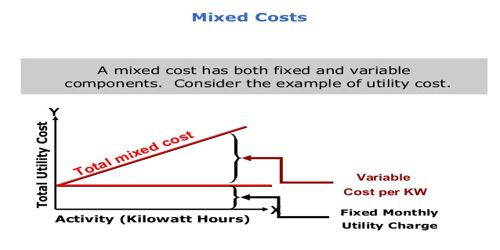Accounting

# Methods of Segregating Mixed CostsMethods of Segregating Mixed Costs

Mixed costs contain elements of both fixed and variable cost behavior. There are numerous methods are used for segregating mixed costs into fixed and variable. Here describe four main techniques that are found in practice and they may be listed as follows:

1. Graphical Method
2. High Points and Low Points Method
3. Analytical Method
4. Least Square Method

Graphical Method: With the graphical method, we draw the graphic line of semi-variable cost by taking output on x’ axis and total semi-variable cost at y’ axis. After this, we do judgment and select a point where will be our fixed cost in semi-variable cost. After this, we draw the line of best fit. This line shows the fixed cost which will not be changed after changing the output.

High Points and Low Points Method: Under this method, we calculate total sale and total cost at the highest level of production. Then we calculate total sale and total cost at the lowest level of production. Because the semi-variable cost has both variable and fixed cost.

Analytical Method: Under this method, the cost accountant does some analysis for dividing the semi-variable cost into fixed cost and variable cost. After this, he calculates the fixed cost on that rate which analyzed. Suppose, a cost accountant says that in the total semi-variable cost, there may be 30% fixed cost and 70% variable cost. Now total semi-variable cost will be divided on this basis. If the production level will increase, variable cost’s proportion will increase at the same rate. But the fixed cost will not change.

Level of Activity Method: In this method, we compare two level of production with the number of expenses in these levels. Variable cost will be calculated with the following method:

Change in semi-variable cost / Change in production volume.

Least Square Method: This is a statistical method to which we use this method for calculating a line of best fit. This method is based on the linear equation y = mx + c, y is the total cost, x is the volume of output and c is total fixed cost. By solving this equation mathematically, we can calculate the variable cost (M) at a different level of production.